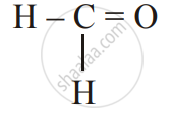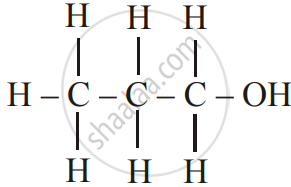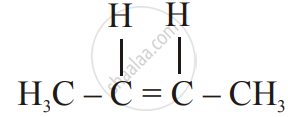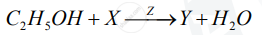Share

Books Shortlist

Chemistry Science paper 2 2017-2018 ICSE Class 10 Question Paper Solution

SubjectChemistry
Year2017 - 2018 (March)
Chemistry
Science paper 2
2017-2018 March
Marks: 80

1
1.1 | Choose the correct answer from the options given below :
1.1.1

The salt solution which does not react with ammonium hydroxide is :

(A) Calcium Nitrate

(B) Zinc Nitrate

(D) Copper Nitrate

Chapter:  Study of Acids, Bases and Salts
Concept: Salt - Types of Salts
1.1.2

The organic compound which undergoes substitution reaction is :

(A) C_2H_2

(B) C_2H_4

(C) C_10H_18

(D) C_2H_6

Chapter:  Organic Chemistry
Concept: Concept of Organic Compounds
1.1.3

The electrolysis of acidified water is an example of :

(A) Reduction

(B) Oxidation

(C) Redox reaction

(D) Synthesis

Chapter:  Electrolysis
Concept: Electrolysis - Electrolytes and Non-Electrolytes
1.1.4

The IUPAC name of dimethyl ether is

(A) Ethoxy methane

(B) Methoxy methane

(C) Methoxy ethane

(D) Ethoxy ethane

Chapter:  Organic Chemistry
Concept: Organic Chemistry - Simple Nomenclature
1.1.5

The catalyst used in the contact process is

(A) Copper

(B) Iron

(D) Manganese dioxide

Chapter:  Organic Chemistry
Concept: Alcohols - Concept of Ethanol
1.2 | Give one word or a phase for the following statements :
1.2.1

The energy released when an electron is added to a neutral gaseous isolated atom to form a negatively charged iron.

Chapter:  Periodic Properties and Variations of Properties:- Physical and Chemical
Concept: Periodic Properties - Electron Affinity
1.2.2

A process of formation of ions from molecules which are not in the ionic state

Chapter:  Periodic Properties and Variations of Properties:- Physical and Chemical
Concept: Periodic Properties - Ionisation Potential
1.2.3

The tendency of an element to form chains of identical atoms.

Chapter:  Organic Chemistry
Concept: Unique Nature of Carbon Atom
1.2.4

The property by which certain hydrated salts, when left exposed to the atmosphere, lose their water of crystallization and crumble into powder.

Chapter:  Study of Acids, Bases and Salts
Concept: General Properties of Salts:
1.2.5

The process by which sulphide ore is concentrated.

Chapter:  Metallurgy
Concept: Occurrence of Metals - Extraction of Metals
1.3
1.3.1

Write balanced chemical equations to show : The oxidizing action of conc. Sulphuric acid on carbon

Chapter: [8.04] Sulphuric Acid
Concept: Sulphuric Acid - Oxidizing Agent When Concentrated
1.3.2

Write a balanced chemical equation for the reaction of sodium hydroxide solution with iron (III) chloride solution

Chapter:  Study of Acids, Bases and Salts
Concept: Concept of Acids and Bases
1.3.3

Write a balanced chemical equation for the action of heat on aluminium hydroxide.

Chapter:  Metallurgy
Concept: Metals and Nonmetals - Concept of Metals and Non-metals
1.3.4

Write a balanced chemical equation for the reaction of zinc with potassium hydroxide solution.

Chapter:  Metallurgy
Concept: Metals and Nonmetals - Concept of Metals and Non-metals
1.3.5

Write a balanced chemical equation for Action of dilute hydrochloric acid on magnesium sulphite.

Chapter:  Study of Acids, Bases and Salts
Concept: General Properties of Salts:
1.4
1.4.1

Give the IUPAC name for of the following :Chapter:  Organic Chemistry
Concept: Organic Chemistry - Simple Nomenclature

Give the IUPAC name for of the followingChapter:  Organic Chemistry
Concept: Organic Chemistry - Simple Nomenclature

Give the IUPAC name for the followingChapter:  Organic Chemistry
Concept: Organic Chemistry - Simple Nomenclature
1.4.2

Write the structural formula of the two isomers of butane

Chapter:  Organic Chemistry
Concept: Organic Chemistry - Simple Nomenclature
1.5
1.5.1

State one relevant observation for the following.

Lead nitrate solution is treated with sodium hydroxide solution dropwise till it is excess.

Chapter:  Analytical Chemistry:- Use of Ammonium Hydroxide and Sodium Hydroxide
Concept: Certain Metals and Their Oxides
1.5.2

State one relevant observation for the following:

At the anode, when molten lead bromide is electrolyzed using graphite electrodes.

Chapter:  Electrolysis
Concept: Electrolysis - Electrolytes and Non-Electrolytes
1.5.3

State your observations when Dilute Hydrochloric acid is added to Lead nitrate solution and the mixture is heated.

Chapter: [8.03] Hydrogen Chloride
Concept: Hydrogen Chloride - Acidic Properties of Its Solution
1.5.4

State one relevant observation for the following:

Anhydrous calcium chloride is exposed to air for some time.

Chapter:  Study of Acids, Bases and Salts
Concept: General Properties of Salts:
1.5.5

State your observations when Barium chloride solution is mixed with Sodium Sulphate Solution.

Chapter:  Analytical Chemistry:- Use of Ammonium Hydroxide and Sodium Hydroxide
Concept: Formation and Colour of Hydroxide Precipitated for Solutions of Salts of Ca, Fe, Cu, Zn and Pb
1.6
1.6.1

Why do ionic compounds have high melting points

Chapter:  Chemical Bonding
Concept: Formation and Properties of Ionic Compounds
1.6.2

Give a reason for Inert gases do not form ions.

Chapter:  Chemical Bonding
Concept: Concept of Coordinate Bond
1.6.3

Give a reason for Ionisation potential increases across a period, from left to right

Chapter:  Periodic Properties and Variations of Properties:- Physical and Chemical
Concept: Periodic Properties - Ionisation Potential
1.6.4

Give a reason for Alkali metals are good reducing agents.

Chapter:  Metallurgy
Concept: Position of the Metals (Alkali Metals and Alkaline Earth Metals) in the Periodic Table
1.6.5

Give a reason for Conductivity of dilute hydrochloric acid is greater than that of acetic acid

Chapter:  Organic Chemistry
Concept: Carboxylic Acids - Concept of Acetic Acid
1.7
1.7.1

Name the gas that is produced in the given cases :

Sulphur is oxidized by concentrated nitric acid.

Chapter: [8.02] Nitric Acid
Concept: Nitric Acid as an Oxidizing Agent.
1.7.2

Name the gas that is produced in the given cases :

An action of dilute hydrochloride acid on sodium sulphide.

Chapter: [8.03] Hydrogen Chloride
Concept: Hydrogen Chloride - Acidic Properties of Its Solution
1.7.3

Name the gas that is produced in the given case:

The action of cold and dilute nitric acid on copper

Chapter: [8.02] Nitric Acid
Concept: Nitric Acid as an Oxidizing Agent.
1.7.4

Name the gas that is produced in each of the given case :

At the anode during the electrolysis of acidified water.

Chapter:  Electrolysis
Concept: Electrolysis - Electrolytes and Non-Electrolytes
1.7.5

Name the gas that is produced in the given case:

The reaction of ethanol and sodium.

Chapter:  Metallurgy
Concept: Metals and Nonmetals - Physical Properties - Metal
1.8 | Fill up the blanks with the correct choice given in brackets.
1.8.1

Ionic or electrovalent compounds do not conduct electricity in their _______ state. (fused/solid)

Chapter:  Chemical Bonding
Concept: Formation and Properties of Ionic Compounds
1.8.2

Electrolysis of aqueous sodium chloride solution will form ________ at the cathode. (Hydrogen gas / Sodium metal)

Chapter:  Electrolysis
Concept: Electrolysis - Electrolytes and Non-Electrolytes
1.8.3

Dry hydrogen chloride gas can be collected by ________ displacement of air. (downward / upward)

Chapter: [8.03] Hydrogen Chloride
Concept: Hydrogen Chloride - Acidic Properties of Its Solution
1.8.4

The most common ore of iron is ________. (Calcium / Haematite)

Chapter:  Metallurgy
Concept: Metals and Their Alloys - Common Ores of Iron, Aluminium and Zinc
1.8.5

The salt prepared by the method of direct combination is _______. (iron (II) chloride / iron (III) chloride)

Chapter:  Study of Acids, Bases and Salts
Concept: General Properties of Salts:
2
2.1

What do you understand by a lone pair of electrons?

Chapter:  Chemical Bonding
Concept: Characteristic Properties of Electrovalent Compounds

Draw the electron dot diagram of Hydionium ion (H = 1; O = 8)

Chapter:  Chemical Bonding
Concept: Characteristic Properties of Electrovalent Compounds
2.2

In Period 3 of the Periodic Table, element B is placed to the left of element A

On the basis of this information, choose the correct word from the brackets to complete the following statements:

1) The element B would have (lower / higher) metallic character than A

2) The element A would probably have (lesser / higher) electron affinity than B.

3) The element A would have (greater / smaller) atomic size than B.

Chapter:  Periodic Properties and Variations of Properties:- Physical and Chemical
Concept: Periodic Properties - Metallic Character
2.3

Copy and complete the following table which refers to the conversion of ions to neutral particles.

 Conversion Ionic equation Oxidation / Reduction Chloride ion to chlorine molecule 1)_________ 2)_________ Lead (II) ion to lead 3)_________ 4)_________
Chapter:  Electrolysis
Concept: Electrolysis - Elementary Study of the Migration of Ions
3
3.1

Write the balanced chemical equation to prepare ammonia gas in the laboratory by using an alkali.

Chapter:  Metallurgy
Concept: Metals and Nonmetals - Concept of Metals and Non-metals

State why concentrated sulphuric acid is not used for drying ammonia gas

Chapter:  Metallurgy
Concept: Metals and Nonmetals - Concept of Metals and Non-metals

Why is ammonia gas not collected over water?

Chapter:  Metallurgy
Concept: Metals and Nonmetals - Concept of Metals and Non-metals
3.2

Name the acid used for the preparation of hydrogen chloride gas in the laboratory. Why is this particular acid preferred to other acids?

Chapter: [8.03] Hydrogen Chloride
Concept: Hydrogen Chloride - Preparation of Hydrogen Chloride from Sodium Chloride

Write the balanced chemical equation for the laboratory preparation of hydrogen chloride gas.

Chapter: [8.03] Hydrogen Chloride
Concept: Hydrogen Chloride - Preparation of Hydrogen Chloride from Sodium Chloride
3.3

For the preparation of hydrochloric acid in the laboratory:

Why is the direct absorption of hydrogen chloride gas in water not feasible?

Chapter: [8.03] Hydrogen Chloride
Concept: Hydrogen Chloride - Preparation of Hydrogen Chloride from Sodium Chloride

For the preparation of hydrochloric acid in the laboratory:

What arrangement is done to dissolve hydrogen chloride gas in water?

Chapter: [8.03] Hydrogen Chloride
Concept: Hydrogen Chloride - Acidic Properties of Its Solution
3.4

For the electro-refining of copper: What is the cathode made up of?

Chapter:  Metallurgy
Concept: Metals and Nonmetals - Concept of Metals and Non-metals

For the electro-refining of copper: Write the reaction that takes place at the anode

Chapter:  Metallurgy
Concept: Metals and Nonmetals - Concept of Metals and Non-metals
4
4.1

The percentage composition of a gas is Nitrogen 82.35%, Hydrogen 17.64%. Find the empirical formula of the gas.  [N = 14, H = 1]

Chapter:  Mole Concept and Stoichiometry
Concept: Mole Concept
4.2

Aluminium carbide reacts with water according to the following equation :

Al_4C_3 + 12H_2O-> 4Al(OH)_3 + 3CH_4

1)What mass of aluminium hydroxide is formed from 12g of aluminium carbide?

2) What volume of methane at s.t.p. is obtained from 12g of aluminium carbide?

[Relatively molecular weight of Al_4Cl_3 = 144; Al(OH)_3 = 78]

Chapter:  Mole Concept and Stoichiometry
Concept: Relative Atomic Masses (Atomic Weight) and Relative Molecular Masses (Molecular Weights)
4.3

If 150 cc of gas A contains X molecules, how many molecules of gas B will be present in 75 cc of B?. The gases A and B are under the same conditions of temperature and pressure.

Chapter:  Mole Concept and Stoichiometry

Name the law om which same conditions of temperature and pressure

Chapter:  Mole Concept and Stoichiometry
4.4

State the main components of the following alloys:

Brass

Chapter:  Metallurgy
Concept: Metals and Their Alloys - Common Ores of Iron, Aluminium and Zinc

State the main components of the following alloys:

Duralumin

Chapter:  Metallurgy
Concept: Metals and Their Alloys - Common Ores of Iron, Aluminium and Zinc
5
5.1

Complete the following table which relates to the homologous series of hydrocarbons.

 General Formula IUPAC name of the homologous series Characteristic bond type IUPAC name of the first member of the series C_nH_(2n-2) (A)________ (B)______ (C)______ C_nH_(2n+1) (B)________ (E)______ (F)______
Chapter:  Organic Chemistry
Concept: Organic Chemistry - Homologous Series
5.2

Name the most common ore of the metal aluminium from which the metal is extracted. Write the chemical formula of the ore.

Chapter:  Metallurgy
Concept: Metals and Their Alloys - Common Ores of Iron, Aluminium and Zinc

Name the process by which impure ore of aluminium gets purified by using the concentrated solution of an alkali.

Chapter:  Metallurgy
Concept: Metals and Their Alloys - Common Ores of Iron, Aluminium and Zinc

Write the equation for the formation of aluminium at the cathode during the electrolysis of alumina.

Chapter:  Metallurgy
Concept: Metals and Their Alloys - Common Ores of Iron, Aluminium and Zinc
6
6.1

A compound X (having the vinegar-like smell) when treated with ethanol in the presence of the acid Z, gives a compound Y which has a fruity smell.

The reaction is:1) Identify Y and Z.

2) Write the structural formula of X.

3) Name the above reaction.

Chapter:  Analytical Chemistry:- Use of Ammonium Hydroxide and Sodium Hydroxide
Concept: Certain Metals and Their Oxides
6.2

Ethane burns in oxygen to form CO2 and H2O according to the equation:

2C_2H_6+7O_2 -> 4CO_2 + 6H_2O

If 1250 cc of oxygen is burnt with 300 cc of ethane.

Calculate:

1) the volume of CO_2 formed

2) the volume of unused O_2

Chapter:  Mole Concept and Stoichiometry
Concept: Deduction of Simple (Empirical) and Molecular Formula from the Percentage Composition of a Compound
6.3

Three solutions P, Q and R have pH value of 3.5, 5.2 and 12.2 respectively. Which one of these is a:

1) Weak acid?

2) Strong alkali?

Chapter:  Study of Acids, Bases and Salts
Concept: Concept of Acids and Bases
7
7.1

Give a chemical test to distinguish between the given pairs of chemicals:

Lead nitrate solution and Zinc nitrate solution

Chapter:  Analytical Chemistry:- Use of Ammonium Hydroxide and Sodium Hydroxide
Concept: Certain Metals and Their Oxides

Give a chemical test to distinguish between the given pairs of chemicals:

Sodium chloride solution and Sodium nitrate solution

Chapter: [8.03] Hydrogen Chloride
Concept: Hydrogen Chloride - Acidic Properties of Its Solution
7.2

Write a balanced equation for the preparation of the following salts:

Copper sulphate from Copper Carbonate.

Chapter:  Study of Acids, Bases and Salts
Concept: General Properties of Salts:

Write a balanced equation for the preparation of the following salts:

Zinc carbonate from Zinc sulphate

Chapter:  Study of Acids, Bases and Salts
Concept: General Properties of Salts:
7.3

What is the type of salt formed when the reactants are heated at a suitable temperature for the preparation of Nitric acid?

Chapter: [8.02] Nitric Acid
Concept: Nitric Acid - Laboratory Method of Preparation of Nitric Acid from Potassium Nitrate Or Sodium Nitrate
7.4

Which property of sulphuric acid is shown by the reaction of the concentrated sulphuric acid with Ethanol?

Chapter: [8.04] Sulphuric Acid
Concept: Sulphuric Acid - Oxidizing Agent When Concentrated

Which property of sulphuric acid is shown by the reaction of the concentrated sulphuric acid with Carbon?

Chapter: [8.04] Sulphuric Acid
Concept: Sulphuric Acid - Oxidizing Agent When Concentrated

Request Question Paper

If you dont find a question paper, kindly write to us

View All Requests

Submit Question Paper

Help us maintain new question papers on shaalaa.com, so we can continue to help students

only jpg, png and pdf files

CISCE previous year question papers Class 10 Chemistry with solutions 2017 - 2018

CISCE Class 10 Chemistry question paper solution is key to score more marks in final exams. Students who have used our past year paper solution have significantly improved in speed and boosted their confidence to solve any question in the examination. Our CISCE Class 10 Chemistry question paper 2018 serve as a catalyst to prepare for your Chemistry board examination.
Previous year Question paper for CISCE Class 10 Chemistry-2018 is solved by experts. Solved question papers gives you the chance to check yourself after your mock test.
By referring the question paper Solutions for Chemistry, you can scale your preparation level and work on your weak areas. It will also help the candidates in developing the time-management skills. Practice makes perfect, and there is no better way to practice than to attempt previous year question paper solutions of CISCE Class 10.

How CISCE Class 10 Question Paper solutions Help Students ?
• Question paper solutions for Chemistry will helps students to prepare for exam.
• Question paper with answer will boost students confidence in exam time and also give you an idea About the important questions and topics to be prepared for the board exam.
• For finding solution of question papers no need to refer so multiple sources like textbook or guides.
S